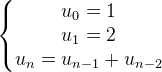# Jonathan Petitcolas

Full-Stack Web Developer, Open-Source Contributor, Seasoned Speaker

# Fibonacci sequence generator in Go

Published on 18 August 2014When completing one of the mathematical challenge of Project Euler, I had to compute some Fibonacci numbers. I found, with the help of François Zaninotto, a very elegant way to get the terms of this famous sequence, based on a StackOverflow answer.

As a reminder, the Fibonacci sequence is defined as:A very naive and simple way to implement it would be the following:

``````func GetFibonacci(first int, second int, rank int) int {
if rank == 1 {
return first
}

if rank == 2 {
return second
}

return GetFibonacci(first, second, rank - 1) + GetFibonacci(first, second, rank - 2)
}
``````

This is just a retranscription of previous formulas. But using Go in this case does not bring anything special. So, here is a more elegant and Go-friendly way, including a generator:

``````func FibonacciGenerator(first int, second int) chan int {
c := make(chan int)

go func() {
for i, j := first, second ; ; j, i = i + j, j {
c <- i
}
}()

return c
}
``````

As the initial conditions may vary, we parametrize the first and second Fibonacci terms. Then, we create a new channel containing only integers with the `make` function. Consider a channel like a FIFO stack: you can insert some values in it with the `->` (move towards) operator or consume them with `<-` (take from). This is the most awesome and damn simple feature of Go language. With channels, you can prevent your code from becoming a callback hell all Node.js developers already experienced.

Then, we declare an anonymous go-routine. A Go routine is simply executed in a separate thread. Just think about it as an asynchronous function. All the Fibonacci logical is located in the `for` clause, using the possibility to assign several variables with a single `=` operator. The following line is the key one: we write `i` value into the channel. Yet, writing into a channel blocks current thread until the value is read. In this case, it simply yield the returned Fibonacci term.

To write a list of 10 first Fibonacci numbers, you can simply use the following code:

``````import "fmt"

// Generator code

func main() {
generator := FibonacciGenerator(1, 1)
for i := 0 ; i < 10 ; i++ {
fmt.Println(<- generator)
}
}
``````

So short but elegant code… The more in Go I develop, the more addicted I become! :)